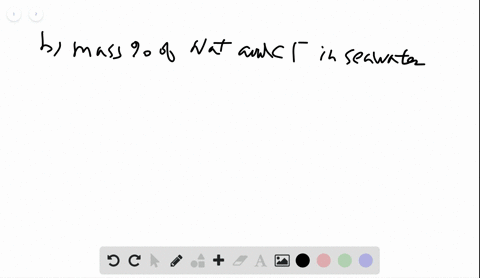Black Friday is Here! Start Your Numerade Subscription for 50% Off!Join Today### Seawater is approximately 4.0$\%$ by mass dissolv…

03:50University of Maine
Problem 112

# The zirconium oxalate $\mathrm{K}_{2} \mathrm{Zr}\left(\mathrm{C}_{2} \mathrm{O}_{4}\right)_{3}\left(\mathrm{H}_{2} \mathrm{C}_{2} \mathrm{O}_{4}\right) \cdot \mathrm{H}_{2} \mathrm{O}$ was synthesized by mixing 1.68 $\mathrm{g}$ of $\mathrm{ZrOCl}_{2} \cdot 8 \mathrm{H}_{2} \mathrm{O}$ with 5.20 $\mathrm{g}$ of$\mathrm{H}_{2} \mathrm{C}_{2} \mathrm{O}_{4} \cdot 2 \mathrm{H}_{2} \mathrm{O}$ and an excess of aqueous $\mathrm{KOH}$ . After 2 months, 1.25 $\mathrm{g}$ of crystalline product was obtained, along with aqueous $\mathrm{KCl}$ and water. Calculate the percent yield.

## Discussion

You must be signed in to discuss.

## Video Transcript

for the following reaction. The first step is to be sure to balance it before looking at calculating the percent yield. When this equation is balanced, the coefficients are one for four, and then the products are one to and 20. It's also helpful before you start to find the molar mass of the compounds involved in the reaction. We find this by adding up the individual molar masses, including the hydrates or the waters using the periodic table. So for the first compound, the molar mass is 322 0.24 grams per mole. My second reacted has a molar mass of 126 0.7 grams per mole. And finally, the product that we form here has a molar mass of 541 0.66 grams per mole. So the question tells us that we start off with an initial amount of both reactive. We have 1.68 grams of the zirconium reactant, and we have five 0.20 grams of the actually reacted, and we know that were produced 1.25 grams of the product to find the percent yield we need to figure out what the theoretical yield is or how much is actually produced, so we need to solve for the theoretical yield or the calculated amount. So to do that, we have both reactors Ingrams, and we change two moles using the molar Mass. And then we have moles of the compound. You changed two moles of the product. We do that by using the balanced equations, the mole ratio from the balanced equation. And then finally, we can change two grams the product using the molar mass of the product. Because we're given both reactions, we need to do this steps for each one and look for which reactant produces the smaller amount. This will be our theoretical yield, so the smaller amount produced is our theoretical yield. Let's start with zirconium complex 1.6 grams, 68 grams to change two moles by dividing by the molar Mass and then, in looking at the balanced equation, receive that it the 1 to 1 ratio between the product and the zirconium, or one mole of product for every one mole of zero r o c. L 2.8 h 20 And then we change from grams, two moles of product multiplying by the molar mass or 541 0.66 grams for every mole of product. Or we produced 2.83 grams. Similarly looking at the second reacted H two C two 04 not to H 20 when we started out with five 0.20 grams, we change it to moles using the molar Mass. 126 0.7 grams. And then, in this case, when we look at the balanced equation, there are formals of reactive for every one mole of product, and then we multiply by 5 41.66 grams per mole where were produced 5.59 grands. This number is the smaller. So that's the theoretical yield to our percent yield is how much we actually produced, which is 1.25 grams, divided by what we should have produced 2.83 grams times 100 or 44 percent